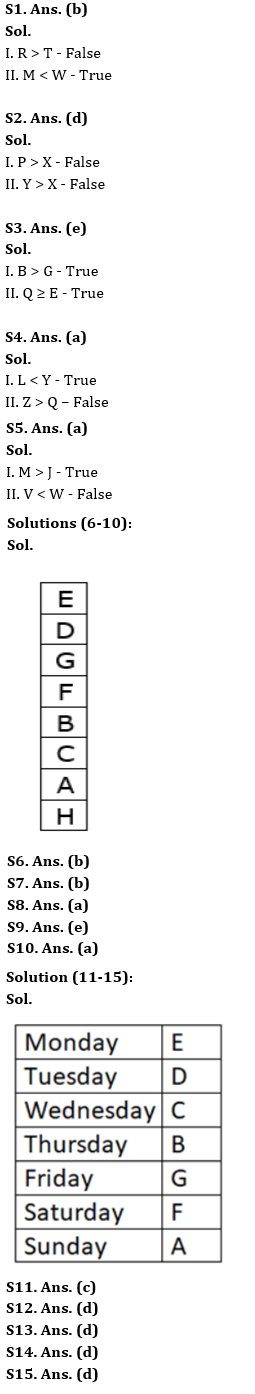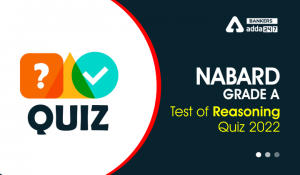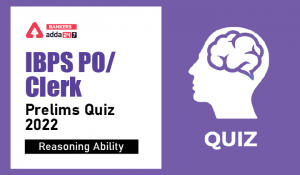Latest Banking jobs   »

# Reasoning Ability Quiz For IBPS Clerk Prelims 2022- 7th July

Directions (1-5): In the given questions, relationship between different elements is shown in the statement(s). These statements are followed by two conclusions. Find which of the conclusion(s) will be definitely true.

Q1.
Statements:
L > M < N = R ≤ S, T ≥ G < W> S
Conclusions:
I. R > T
II. M < W
(a) Only conclusion I is true
(b) Only conclusion II is true
(c) Either conclusion I or II is true
(d) Neither conclusion I nor II is true
(e) Both conclusions I and II are true

Q2.
Statements:
P ≥ Q, Q ≤ X, X > S, S < Y
Conclusions:
I. P > X
II. Y > X
(a) Only conclusion I is true
(b) Only conclusion II is true
(c) Either conclusion I or II is true
(d) Neither conclusion I nor II is true
(e) Both conclusions I and II are true

Q3.
Statements:
N = K < E ≤ B = Q ≥ U > G
Conclusions:
I. B > G
II. Q ≥ E
(a) Only conclusion I is true
(b) Only conclusion II is true
(c) Either conclusion I or II is true
(d) Neither conclusion I nor II is true
(e) Both conclusions I and II are true

Q4.
Statements:

L ≤ Q, N > Z, L ≤ Z, N = Y
Conclusions:
I. L < Y
II. Z > Q
(a) Only conclusion I is true
(b) Only conclusion II is true
(c) Either conclusion I or II is true
(d) Neither conclusion I nor II is true
(e) Both conclusions I and II are true

Q5.
Statements:
W ≥ U = J, K ≤ V = S, S < M > W
Conclusions:
I. M > J
II. V < W
(a) Only conclusion I is true
(b) Only conclusion II is true
(c) Either conclusion I or II is true
(d) Neither conclusion I nor II is true
(e) Both conclusions I and II are true

Directions (6-10): Study the following information carefully and answer the given questions.
Eight boxes i.e. A, B, C, D, E, F, G, and H are placed one above the other in a stack but not necessarily in the same order. Two boxes are placed between Box H and Box B. Box H is either placed at topmost or at bottommost position. Three boxes are placed between Box C and Box D which is placed above box B. One box is placed between Box D and Box F. One box is placed between Box E and Box G which is placed above Box A. More than three boxes are placed between Box A and Box E.

Q6. Which box is placed at 6th position from bottom?
(a) D
(b) G
(c) F
(d) B
(e) None of these

Q7. How many boxes are placed below Box A?
(a) Three
(b) One
(c) None
(d) Two
(e) More than three

Q8. How many boxes are placed between Box G and Box A?
(a) Three
(b) More than three
(c) None
(d) Two
(e) One

Q9. Four of the following five pairs are alike in a certain way and hence form a group. Find the one which does not belong to that group.
(a) H-A
(b) F-G
(c) D-E
(d) C-A
(e) G-H

Q10. If we arrange all the boxes according to English alphabetical series from bottom to top, then how many boxes will remain at same place?
(a) One
(b) Three
(c) Two
(d) Five
(e) None

Directions (11-15): Study the following information carefully and answer the given questions.
Seven friends A, B, C, D, E, F, and G visit a museum on seven different days of the same week starting from Monday. Only two of them visit the museum between B and E. Two persons visit the museum between A and B. D visits the museum immediately before C but not on Friday. G does not visit on Saturday. Less than four of them visit the museum between A and F.

Q11. Who visits the museum on Sunday?
(a) G
(b) F
(c) A
(d) D
(e) None of these

Q12. How many of them visit the museum between C and F?
(a) Four
(b) One
(c) Three
(d) Two
(e) None of these

Q13. G visits the museum on which of the following days?
(a) Tuesday
(b) Monday
(c) Thursday
(d) Friday
(e) None of these

Q14. Four among the following five pairs are alike in a certain way and forms a group. Which among the following does not belong to the group?
(a) D, B
(b) A, G
(c) G, C
(d) B, E
(e) F, B

Q15. The number of people visits the museum after G is the same as the number of people visits the museum before ____.
(a) F
(b) E
(c) D
(d) C
(e) None of the above

Solutions#### Congratulations!Incorrect details? Fill the form again here

•Reasoning Ability Quiz For IBPS RRB PO C...
•Reasoning Ability Quiz For NABARD Grade ...
•Reasoning Ability Quiz For IBPS Clerk/PO...
•Reasoning Ability Quiz For IBPS RRB PO C...
•Reasoning Ability Quiz For IBPS RRB PO C...
•Reasoning Ability Quiz For IBPS Clerk Pr...